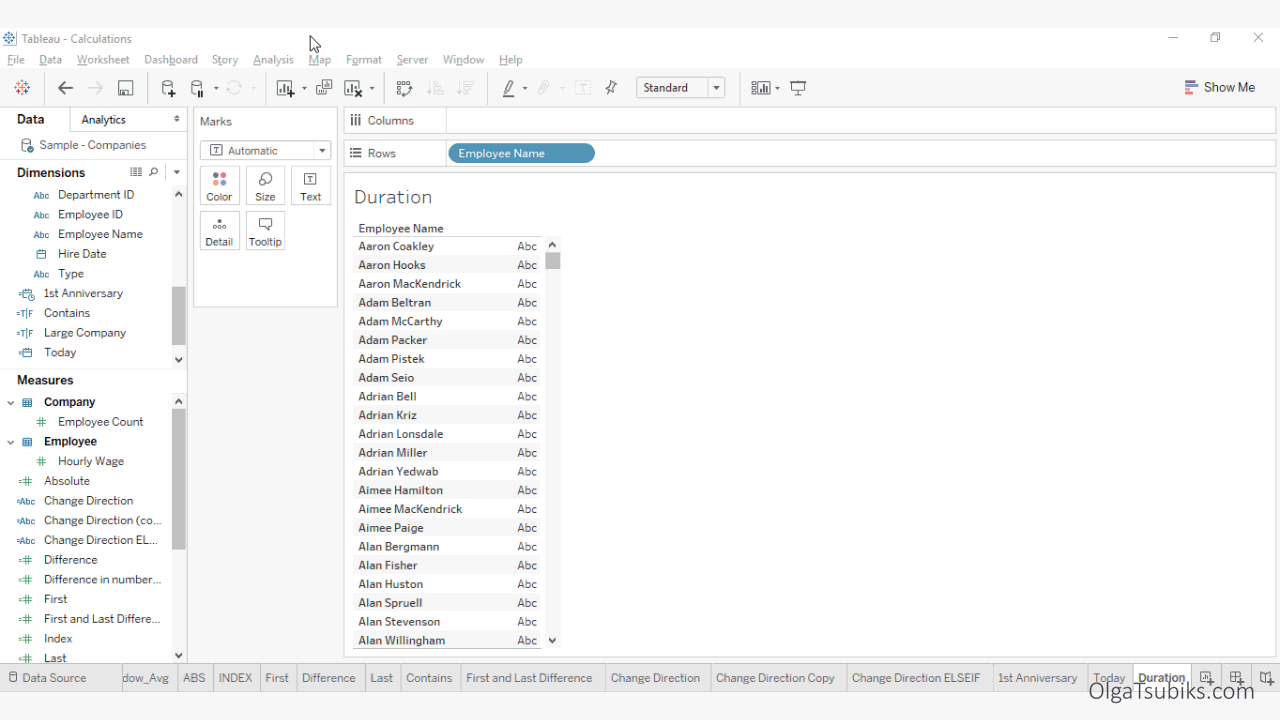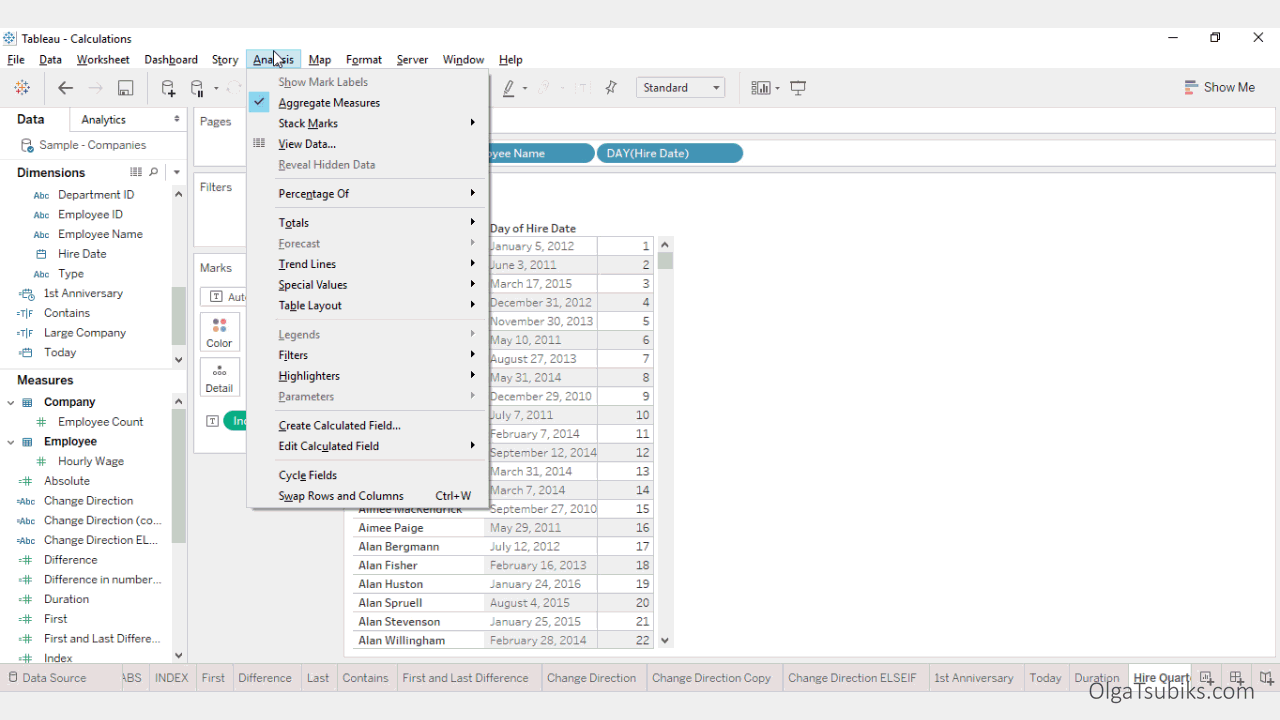PART VIII

## Date Calculations in Tableau

This returns the specified date, with the specified number interval added to the specified date part of that date.

For example, let's find out the date when each employee would have their 1-year-with-the-company anniversary.Solution: Create a new calculation called 1st Anniversary. Add the following calculation: DATEADD('year', 1, [Hire Date]). Add the calculation to the Columns Shelf to view the result. For example, Aaron Hooks's 1st anniversary is on June 3, 2012.

## TODAY()

This returns the current date.

For example, let's create a new calculation called Today.Solution:

Create a new calculation called Today. Add the following calculation: TODAY().

## DATEDIFF(date_part, date1, date2, [start_of_week])

This returns the difference between date1 and date2, expressed in units of date_part. The start_of_week parameter is optional.

For example, let's calculate how many years have passed since the hire date.Solution: Create a new calculation called Duration. Add the following calculation: DATEIFF('year', [Hire Date], [Today]).

For example, Aaron Hooks has been with the company for 6 years.

## DATETRUNC(date_part, date, [start_of_week])

This truncates the specified date to the accuracy specified by the date_part. This function returns a new date.

For example, let's find the first date of the quarter in which the employee was hired.Solution: Create a new calculation called Hire Quarter. Add the following calculation: DATETRUNC('quarter', [Hire Date]). For example, the first day of the quarter in which Aaron Hooks was hired is April 1, 2011.

## DATEPART(date_part, date, [start_of_week])

This returns the date_part of the date as an integer. The start_of_week parameter is optional.

For example, let's get the day of the date on which each employee was hired.Solution:

Create a new calculation called Hire Day. Add the following calculation: DATEPART('day', [Hire Date]).